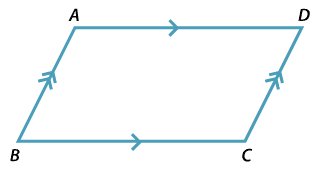##### Definition of a parallelogram

A parallelogram is a quadrilateral whose opposite sides are parallel.

#### Properties of a parallelogram

• The opposite angles of a parallelogram are equal.
• The opposite sides of a parallelogram are equal.
• The diagonals of a parallelogram bisect each other.

#### Tests for a parallelogram

A quadrilateral is a parallelogram if:

• its opposite angles are equal, or
• its opposite sides are equal, or
• one pair of opposite sides are equal and parallel, or
its diagonals bisect each other.